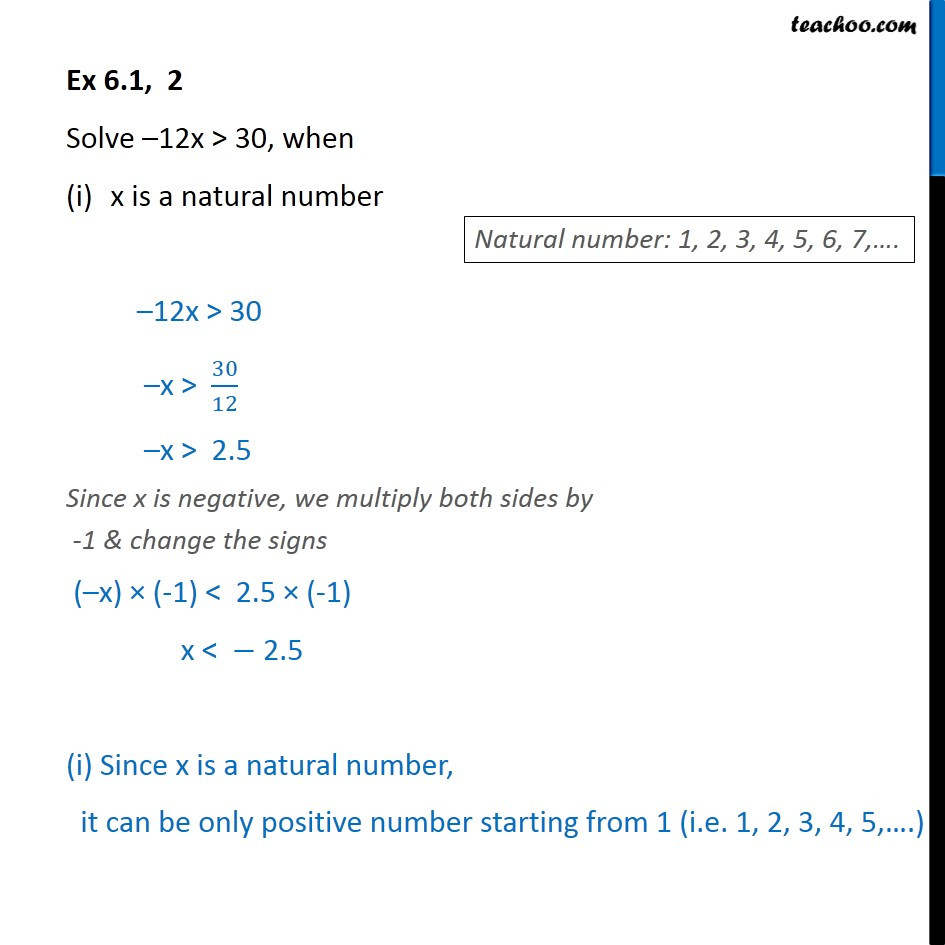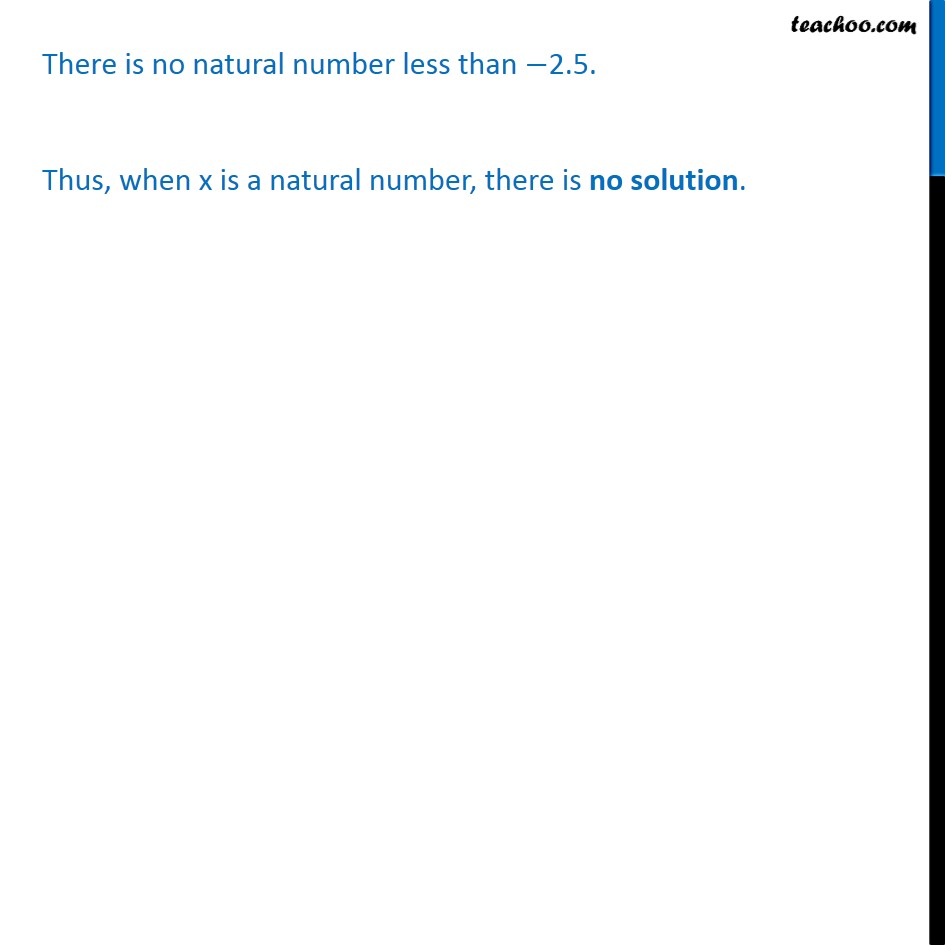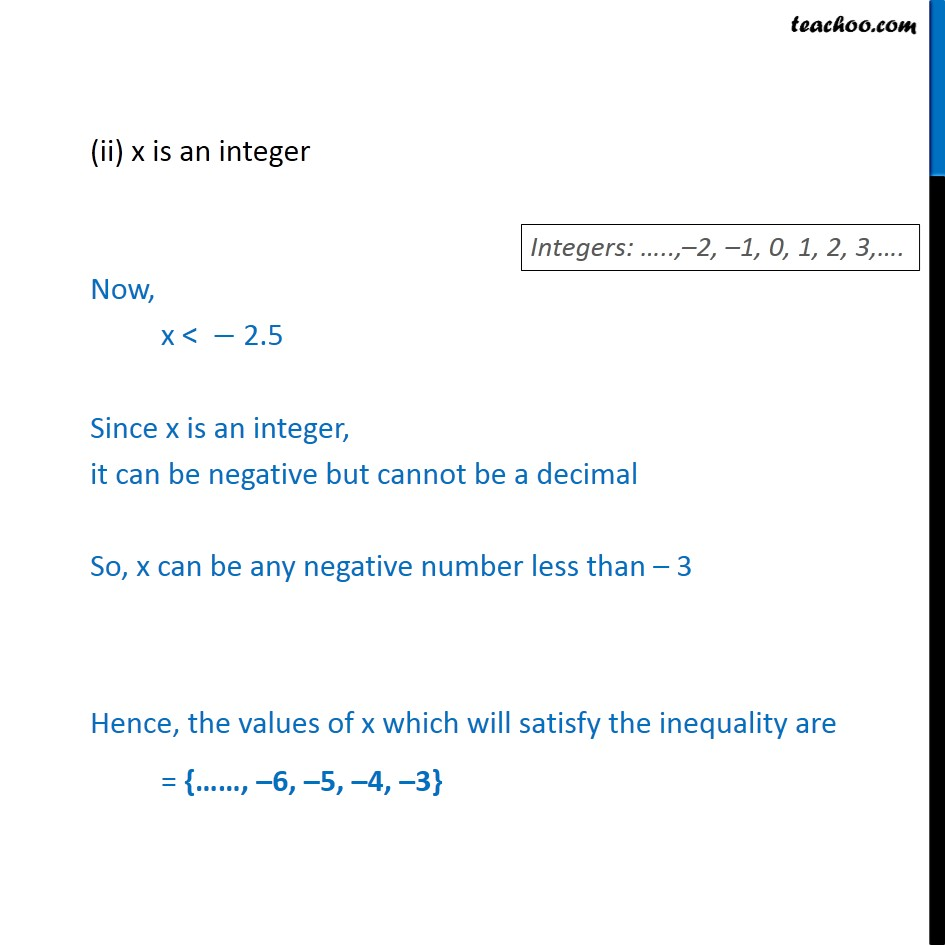Ex 6.1

Chapter 6 Class 11 Linear Inequalities (Term 2)
Serial order wise### Transcript

Ex 6.1, 2 Solve 12x > 30, when x is a natural number 12x > 30 x > 30 12 x > 2.5 Since x is negative, we multiply both sides by -1 & change the signs ( x) (-1) < 2.5 (-1) x < 2.5 (i) Since x is a natural number, it can be only positive number starting from 1 (i.e. 1, 2, 3, 4, 5, .) There is no natural number less than 2.5. Thus, when x is a natural number, there is no solution. (ii) x is an integer Now, x < 2.5 Since x is an integer, it can be negative but cannot be a decimal So, x can be any negative number less than 3 Hence, the values of x which will satisfy the inequality are = { , 6, 5, 4, 3}